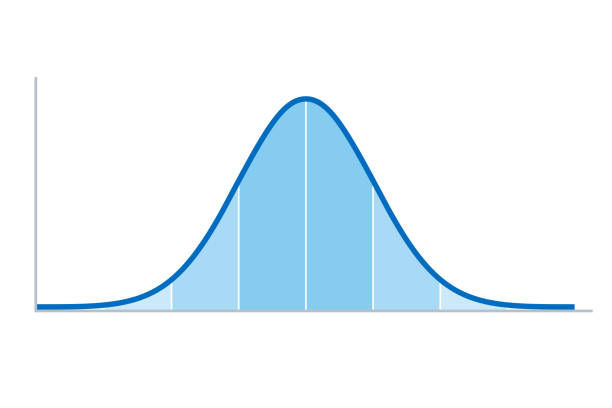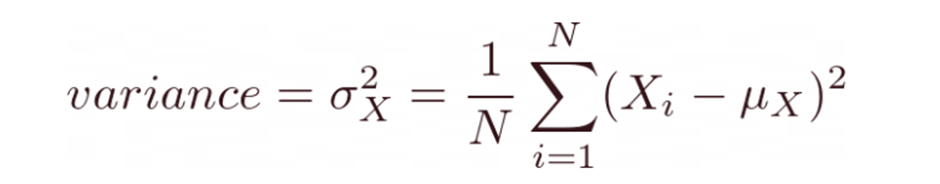top of pageSearch

# Spread and variance in statistics### Variability

Variability describes how far apart data points lie from each other and from the center of a distribution. Along with measures of central tendency, measures of variability give you descriptive statistics that summarize your data.

While the central tendency, or average, tells you where most of your points lie, variability summarizes how far apart they are. This is important because the amount of variability determines how well you can generalize results from the sample to your population.

Low variability is ideal because it means that you can better predict information about the population based on sample data. High variability means that the values are less consistent, so it’s harder to make predictions.

Measures of spread describe how similar or varied the set of observed values are for a particular variable (data item). Measures of spread include the range, quartiles and the interquartile range, variance and standard deviation.

The spread of the values can be measured for quantitative data, as the variables are numeric and can be arranged into a logical order with a low end value and a high end value.

Data sets can have the same central tendency but different levels of variability or vice versa. If you know only the central tendency or the variability, you can’t say anything about the other aspect. Both of them together give you a complete picture of your data.

Let us now learn about the spread and variance with an example of heights of students in 2 rows.

The figure below shows the heights of students of top rowThe average value for height of the students is 122.3

The figure below shows heights of students in bottom rowIf we find the average height of the students here, it will be 122.05.

Though the average heights in case of both the rows were very similar, we can visually see that there is more difference in heights around the mean height in the top row compared to the bottom row.

The figure below shows the position of the height values in the top row with respect to the mean value (indicated using dashed line)Similarly the below figure shows the position of the height values in the bottom row with respect to the mean value (indicated using broken line)#### Variance and standard deviation

The variance and the standard deviation are measures of the spread of the data around the mean. They summarize how close each observed data value is to the mean value. In datasets with a small spread all values are very close to the mean, resulting in a small variance and standard deviation. Where a dataset is more dispersed, values are spread further away from the mean, leading to a larger variance and standard deviation. The smaller the variance and standard deviation, the more the mean value is indicative of the whole dataset. Therefore, if all values of a dataset are the same, the standard deviation and variance are zero.

To find the variance, we find the average of the squares of the difference of each point from the mean.

In the above example, the variance for heights in the top row is 106.0 but for the bottom row, the variance is 12.7.

The value for the variance for the bottom row (=12.7) is significantly smaller than the value for the variance for the top row (=106).

In general for an ordered list of values XX with NN elements, the variance can be obtained using the below formula,The heights of the kids in both the rows can be summarized in the following histogramsFrom the histograms above, it’s very clear that the height values were spread over more bins in case of the top row as compared to the bottom row. This is indicative of higher variance in top row as compared to the bottom row

Variance captures the spread of the data around the mean value. In the above histograms the dashed lines indicate the value of the mean.

Histograms are a very useful visual aid to understand the spread of data, especially when comparing two different similar sets of data

Another widely used measure to quantify the spread around the mean value is called the Standard Deviation (SD). Conveniently, SD is just the square root of the variance. In order to calculate the standard deviation, all one has to do is to calculate the variance and then find the square root of the variance !### Conclusion

We have learnt the meaning of variability, measure of spread, variance and standard deviation